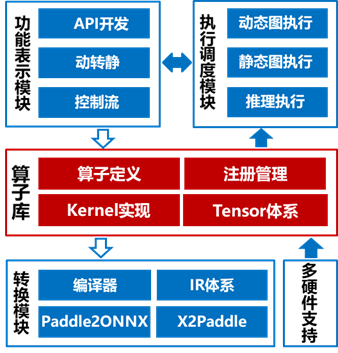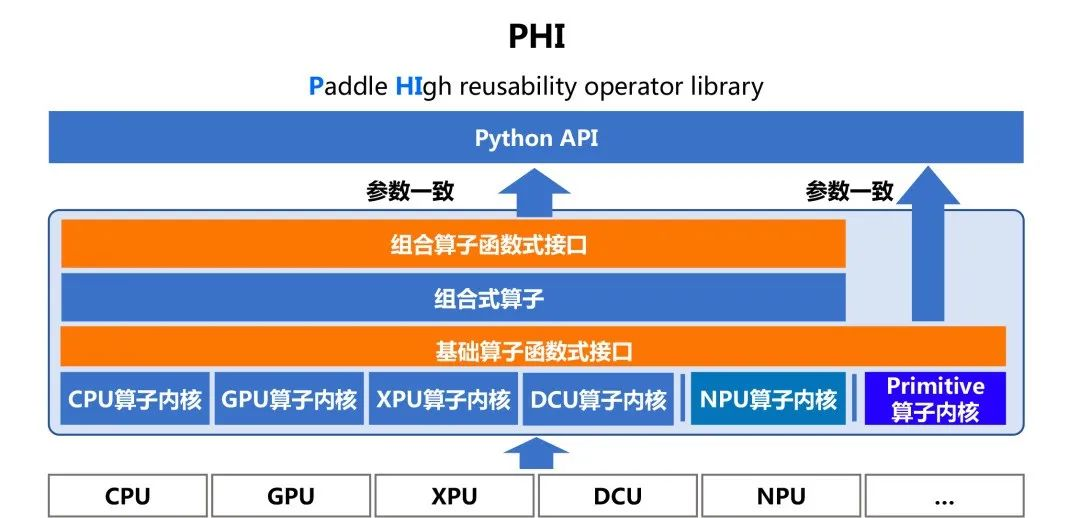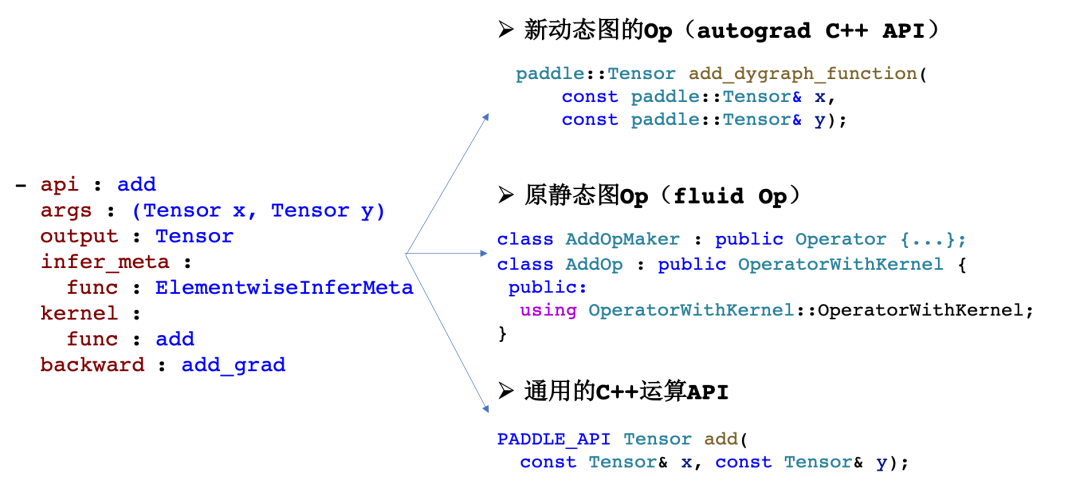\u200EPHI架构简介

PHI算子库采用多层设计。最下层是基础算子的函数式接口和与之相对应的各个硬件的内核实现，包含已经支持的CPU内核、GPU内核及XPU内核；同时，与之前已经发布的Primitive内核体系也进行了适配。在基础算子体系之上，可以通过组合的方式实现复杂算子，并声明相应的函数式接口，目前组合式算子仍在逐步建设中。对于函数式接口的命名和参数列表，在设计上与Python端相应API保持一致，以降低开发者的学习成本。PHI算子库致力于降低算子开发成本和新硬件接入成本，为此升级完善了多项核心机制。

• 配置式算子定义 采用基于yaml配置的算子定义方式，替换掉了原先基于C++类的算子定义方式，写法上更加简洁清晰，同时解耦框架实现也提升了灵活性。
• 函数式算子内核 ：支持通过调用函数的方式复用基础算子内核来组合开发更复杂的算子内核。例如，通过对矩阵乘、加减乘除法等基础算子函数式接口的调用，很容易实现一个Linear或者SGD算子内核，高效支撑算子开发需求。以复杂的Einsum算子为例，基于PHI算子库进行组合式实现，所需代码量相比从零开始实现能够显著减少，开发成本显著降低。
• 基于C++运算API的插件式算子开发 ：我们将之前发布的自定义算子机制与PHI算子库进行了整合，支持在开发自定义算子时使用PHI提供的C++运算API，有效降低了外部算子的开发成本。

• 插件式算子内核开发 ：我们支持在外部实现特定硬件的算子内核，与框架实现解耦，以插件的形式接入框架使用，支持低成本接入硬件或相应加速库。
• Primitive内核接口体系 ：支持不同芯片之间的算子内核复用代码实现，提升算子内核的开发效率。例如，基于Primitive接口的算子内核的复用，能够使用同一份代码支持GPU和XPU硬件，有效降低了新硬件适配算子的成本。

（1）简洁性

PHI算子库的算子定义方式相比之前有较大的升级。以Transpose为例进行对比，原来的算子定义方式是基于类进行实现，新增TransposeOp类，实现维度推导类方法，同时新增TransposeOpMaker，定义算子的输入、输出和属性；而新的PHI算子定义方式整体是配置式的，只需要像“完型填空”一样，将关键的信息填写到对应条目之后即可。通过对比，可以看出新的算子定义方式无论是简洁性还是清晰度相比之前都有较大的改善。

``// 原来的算子定义方式class TransposeOp : public framework::OperatorWithKernel { public:  using framework::OperatorWithKernel::OperatorWithKernel;  void InferShape(framework::InferShapeContext *ctx) const override {...}};class TransposeOpMaker : public framework::OpProtoAndCheckerMaker { public:  void Make() override {    AddInput(        "X",        "(Tensor) The input tensor, tensors with rank up to 6 are supported.");    AddOutput("Out", "(Tensor)The output tensor.");    AddAttr<std::vector<int>>(        "axis",        "(vector<int>) A list of values, and the size of the list should be "        "the same with the input tensor rank. This operator permutes the input "        "tensor's axes according to the values given.");    AddComment(R"DOC(Transpose Operator.)DOC");  }};// PHI算子定义方式- api : transpose  args : (Tensor x, int[] axis)  output : Tensor  infer_meta :    func : TransposeInferMeta  kernel :    func : transpose  backward : transpose_grad``

（2）灵活性（3）复用性优势

```

`- api : conj  args : (Tensor x)  output : Tensor  infer_meta :    func : UnchangedInferMeta  kernel :    func : conj  backward : conj_grad  - api : atan  args : (Tensor x)  output : Tensor  infer_meta :    func : UnchangedInferMeta  kernel :    func : atan  backward : atan_grad`
```

```

`- api : zeros_like  args : (Tensor x, DataType dtype=DataType::UNDEFINED, Place place = {})  output : Tensor  invoke : full_like(x, 0, dtype, place)`
```

（1）学习理解成本低

“函数”是基本的编程范式，是广大开发者都熟悉的概念，“函数”的输入、计算、输出均有直观清晰的表示，相较于仿函数类+Context输入的形式理解门槛较低。对比示例如下：

• 仿函数类+Context输入形式内核

```

`template <typename DeviceContext, typename T>class TransposeKernel : public framework::OpKernel<T> { public:  void Compute(const framework::ExecutionContext& context) const override {    auto* x = context.InputVar("X");    auto* out = context.OutputVar("Out");    std::vector<int> axis = context.Attr<std::vector<int>>("axis");    // Kernel Implementation  }};`
```
• 函数式内核

```

`template <typename T, typename Context>void TransposeKernel(const Context& ctx,                     const DenseTensor& x,                     const std::vector<int>& axis,                     DenseTensor* out) {  // Kernel Implementation}`
```

（2）复用便捷

y=x2

dx=dy * (2 * x)

``// square前向内核实现及注册template <typename T, typename Context>void SquareKernel(const Context& dev_ctx,                  const DenseTensor& x,                  DenseTensor* out) {  PowKernel<T>(dev_ctx, x, 2, out);}PD_REGISTER_KERNEL(    square, CPU, ALL_LAYOUT, phi::SquareKernel, float, double, int, int64_t) {}// square反向内核实现及注册template <typename T, typename Context>void SquareGradKernel(const Context& dev_ctx,                      const DenseTensor& x,                      const DenseTensor& out_grad,                      DenseTensor* x_grad) {  MultiplyKernel<T>(dev_ctx,                    out_grad,                    Multiply<T>(dev_ctx, Full<T>(dev_ctx, {1}, 2.0), x),                    x_grad);}PD_REGISTER_KERNEL(square_grad,    CPU, ALL_LAYOUT, phi::SquareGradKernel, float, double, int, int64_t) {}``

（3）复用性能高

``// 动态内核分发A() {  判断满足CPU执行条件：// 运行时判断    全局映射表中查找是否存在A_CPU()内核；// 运行时查找    找到A_CPU()并调用  判断满足GPU执行条件：    全局映射表中查找是否存在A_CPU()内核；    找到A_CPU()并调用}// 静态内核分发// 编译时生成了A<CPU>和A<GPU>的函数内核，通过实际Device模板参数匹配所需内核，无需运行时判断查找A<Device>()``

``PADDLE_API Tensor abs(const Tensor& x);PADDLE_API Tensor acos(const Tensor& x);PADDLE_API Tensor acosh(const Tensor& x);PADDLE_API Tensor add(const Tensor& x, const Tensor& y);...``

``std::vector<paddle::Tensor> PhiLinearForward(const paddle::Tensor& x,                                             const paddle::Tensor& weight,                                             const paddle::Tensor& bias) {  return {paddle::add(paddle::matmul(x, weight), bias)};}``
Linear的反向逻辑会略微复杂一些。 因为Linear的前向是有三个输入，对应反向的时候会有三个输出，这三个输出都要独立计算出结果，计算逻辑介绍及示例如下：

``std::vector<paddle::Tensor> PhiLinearBackward(const paddle::Tensor& x,                                              const paddle::Tensor& weight,                                              const paddle::Tensor& bias,                                              const paddle::Tensor& out_grad) {  auto x_grad = paddle::matmul(out_grad, weight, false, true);  auto flatten_x = paddle::reshape(x, {-1, weight.shape()});  auto flatten_out_grad = paddle::reshape(out_grad, {-1, weight.shape()});  auto weight_grad = paddle::matmul(flatten_x, flatten_out_grad, true, false);  std::vector<int64_t> axis;  for (size_t i = 0; i+1 < x.shape().size(); ++i) {    axis.emplace_back(i);  }  auto bias_grad = paddle::experimental::sum(out_grad, axis);  return {x_grad, weight_grad, bias_grad};}``